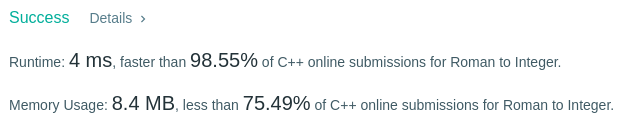# Coding Spirit

0%

Algorithm Puzzles everyday every week sometimes: Roman to Integer

## Puzzle

Puzzle from leetcode:
Roman numerals are represented by seven different symbols: I, V, X, L, C, D and M.

For example, two is written as II in Roman numeral, just two one’s added together. Twelve is written as, XII, which is simply X + II. The number twenty seven is written as XXVII, which is XX + V + II.

Roman numerals are usually written largest to smallest from left to right. However, the numeral for four is not IIII. Instead, the number four is written as IV. Because the one is before the five we subtract it making four. The same principle applies to the number nine, which is written as IX. There are six instances where subtraction is used:

I can be placed before V (5) and X (10) to make 4 and 9.

X can be placed before L (50) and C (100) to make 40 and 90.

C can be placed before D (500) and M (1000) to make 400 and 900.

Given a roman numeral, convert it to an integer. Input is guaranteed to be within the range from 1 to 3999.

## Solution

Result:

Runtime beats 98.55% in all cpp submissions: